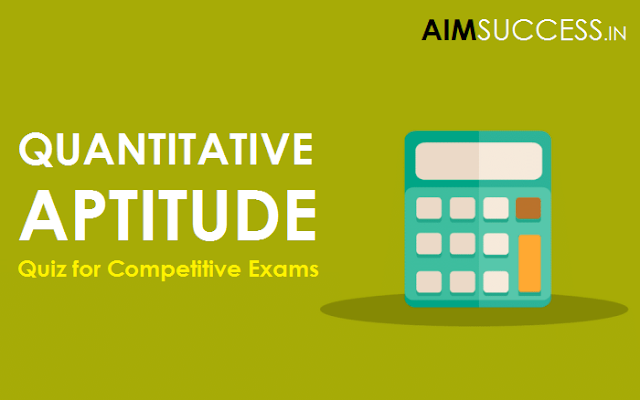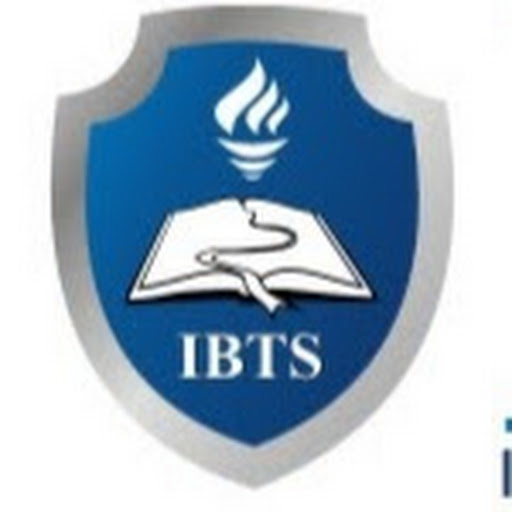# Quantitative Aptitude Quiz for IBPS PO/RRB

##Quantitative Aptitude Quiz for IBPS PO/RRB

1. An article was purchased for Rs. 78,350. Its price was marked up by 30%. It was sold at a discount of 20% on the marked-up price. What was the profit per cent on the cost price?
1.  4%
2.  7%
3.  5%
4.  3%
5.  6%
2. The sum of three consecutive numbers is 2262. What is 41% of the highest number?
1.  301.51
2.  303.14
3.  308.73
4.  306.35
5.  309.55
3. The fare of a bus is Rs. X for the first five kilometres and Rs. 13 per kilometre thereafter. If a passenger pays Rs. 2402 for a journey of 187 kilometres, what is the value of X?
1.  Rs. 29
2.  Rs. 39
3.  Rs. 36
4.  Rs. 31
5.  None of these
4.  The area of a square is 1444 square metres. The breadth of a rectangle is 1/4 the side of the square and the length of the rectangle is thrice its breadth. What is the difference between the area of the square and the area of the rectangle?
1.  1152.38 sq mtr
2.  1169.33 sq mtr
3.  1181.21 sq mtr
4.  1173.25 sq mtr
5.  None of these
5.  A and B together can complete a task in 20 days. B and C together can complete the same task in 30 days. A and C together can complete the same task in 40 days. What is the ratio of the number of days taken by A when completing the same task alone to the number of days taken by C when completing the same task alone ?
1.  2 : 5
2.  2 : 7
3.  3 : 7
4.  1 : 5
5.  3 : 5
6. In an examination, it is required to get 296 marks out of the aggregate marks to pass. A student gets 222 marks and is declared failed by 10% marks. What is the maximum aggregate marks a student can get?
1.  830
2.  810
3.  780
4.  760
5.  None of these
7. A and B started a business with Rs. 3000 and Rs. 4000 respectively. After eight months A withdraws Rs. 1000 and B invests Rs.  1000 more. At the end of the year, their profits amounted to Rs. 630. Then the share of A is
1. Rs.  180
2. Rs.  360
3. Rs.  200
4. Rs.  240
5. None of these
Directions (Q. 8-10): Study the information given below and answer the questions that follow:
An article was bought for Rs. 5600. Its price was marked up by 12%. Thereafter it was sold at a discount of 5% on the marked price.
8. What was the marked price of the article?
1. Rs.  6207
2. Rs.  6242
3. Rs.  6292
4. Rs.  6192
5. Rs.  6272
9. What was the per cent profit on the transaction?
1.  6.8%
2.  6.3%
3.  6.4%
4.  6.6%
5.  6.2%
10. What was the amount of discount given?
1. Rs.  319.6
2. Rs.  303.6
3. Rs.  306.3
4. Rs.  313.6
5. Rs.  316.9
1. 1
2. 5
3. 3
4. 4
5. 4
6. 5
7. 4
8. 5
9. 3
10. 4
###### Share To:### IBTS INDIA

IBTS - (Institute for Banking Training & Educational Services) is in the Leading Institution for banking & SSC, IBPS, Center & State exams in Chandigarh.